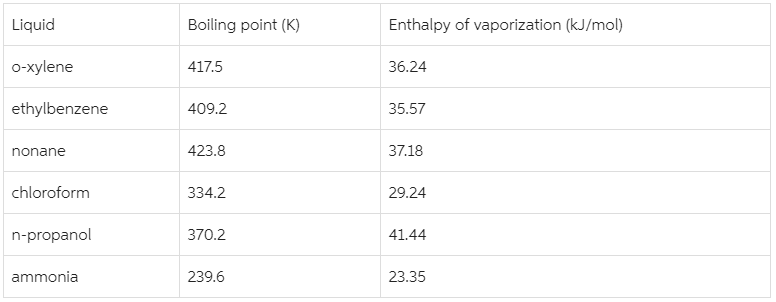Chemistry Practice Problems Entropy Practice Problems Solution: Predict which of the following compounds follow Tr...

🤓 Based on our data, we think this question is relevant for Professor Ratliff's class at USF.

# Solution: Predict which of the following compounds follow Trouton's rule:

###### Problem

Predict which of the following compounds follow Trouton's rule:###### Solution

We have to determine which of the liquids follow Trouton’s rule.

Trouton’s rule states that the entropy of vaporization for many liquids is in the range of 85-88 J/mol•K.

To find out which of the given liquids follow Trouton’s rule, we will calculate the entropy of vaporization for each of the liquid using the given enthalpy and boiling boiling.

We will use this formula:View Complete Written Solution

Entropy

Entropy

#### Q. The element gallium (Ga) freezes at 29.8°C, and its molar enthalpy of fusion is ΔH fus = 5.59kJ/mol.1. When molten gallium solidifies to Ga(s) at its ...

Solved • Wed Jul 10 2019 19:01:42 GMT-0400 (EDT)

Entropy

#### Q. For each of the following pairs, predict which substance possesses the larger entropy per mole.Compare 1 mol of O2(g)  at 300 oC, 0.01 atm and 1 mol o...

Solved • Thu Jan 03 2019 09:45:17 GMT-0500 (EST)

Entropy

#### Q. In chemical kinetics, the entropy of activation is the entropy change for the process in which the reactants reach the activated complex.Predict whe...

Solved • Thu Jan 03 2019 09:45:17 GMT-0500 (EST)

Entropy

#### Q. For each of the following pairs, predict which substance has the higher entropy per mole at a given temperature.He(l) or He(g).

Solved • Thu Jan 03 2019 09:45:16 GMT-0500 (EST)